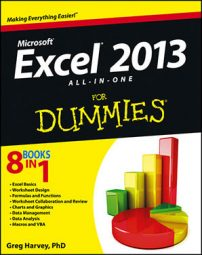##### Excel 2013 All-in-One For DummiesAll the hot key sequences for selecting the most common formula-related commands in Excel 2013 begin with the sequence Alt+M — the M in forMulas was the only mnemonic still available. After you know to press Alt+M to access one of the commands on the Formula tab of the Ribbon, it's pretty easy sailing, if for no other reason than the commands on the Formula tab are pretty evenly and logically laid out.

Hot Keys Excel Ribbon Command Function
Alt+MF Formulas→Insert Function Opens the Insert Function dialog box (same as clicking the Insert Function button on the Formula bar)
Alt+MUS Formulas→AutoSum→Sum (Alt+=) Selects the occupied range above the cell cursor and inserts SUM formula to total the range
Alt+MUA Formulas→AutoSum→Average Selects the occupied range above the cell cursor and inserts AVERAGE formula to calculate the average of total in the range
Alt+MUC Formulas→AutoSum→Count Numbers Selects the occupied range above the cell cursor and inserts COUNT formula to count the number of values in the range
Alt+MI Formulas→Financial Opens a drop-down menu listing all Financial functions — click name to insert function into current cell
Alt+ME Formulas→Date & Time Opens a drop-down menu listing all Date and Time functions — click name to insert function into current cell
Alt+MN Formulas→Name Manager Opens the Name Manager dialog box showing all range names in the workbook, where you can add, edit, and delete names
Alt+MMD Formulas→Define Name Opens New Name dialog box, where you can assign a name to the cell selection or define a new constant
Alt+MS Formulas→Use in Formula Displays drop-down menu with range names in the workbook that you can insert into current formula by clicking
Alt+MC Formulas→Create from Selection Opens Create Names from Selection dialog box, where you indicate which rows and columns to use in naming cell selection
Alt+MH Formulas→Show Formulas (Ctrl+`) Displays and then hides all formulas in cells of the worksheet
Alt+MXA Formulas→Options→Automatic Turns automatic recalculation back on
Alt+MXE Formulas→Options→Automatic Except for Data Tables Turns automatic recalculation back on for all parts of the worksheet except for ranges with Data Tables
Alt+MXM Formulas→Options→Manual Turns on manual recalculation
Alt+MB Formulas→Calculate Now (F9) Recalculates formulas throughout the entire workbook when manual recalculation is turned on
Alt+MJ Formulas→Calculate Sheet (Shift+F9) Recalculates formulas in the current worksheet when manual recalculation is turned on